# The while Statement while boolean expression statement Boolean

• Slides: 15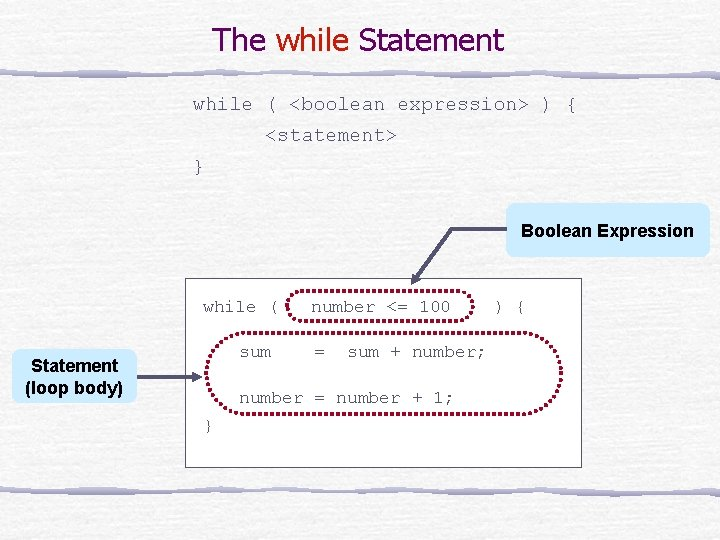The while Statement while ( <boolean expression> ) { <statement> } Boolean Expression while ( sum Statement (loop body) number <= 100 = sum + number; number = number + 1; } ) {Control Flow of while int sum = 0, number = 1 number <= 100 ? false true sum = sum + number; number = number + 1;Example Programs Puurginooo … out to reality … While 10000. java Puurginooo … out to reality … While. Input. java Puurginooo … out to reality … While. Boolean. javawhile Loop Pitfall - 1 1 int product = 0; while ( product < 500000 ) { product = product * 5; } Infinite Loops 2 int count = 1; while ( count != 10 ) { count = count + 2; } Both loops will not terminate because the boolean expressions will never become false.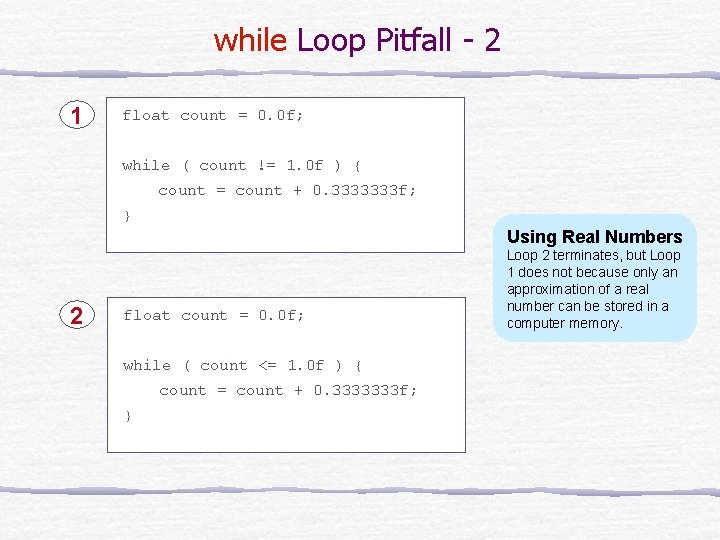while Loop Pitfall - 2 1 float count = 0. 0 f; while ( count != 1. 0 f ) { count = count + 0. 3333333 f; } Using Real Numbers 2 float count = 0. 0 f; while ( count <= 1. 0 f ) { count = count + 0. 3333333 f; } Loop 2 terminates, but Loop 1 does not because only an approximation of a real number can be stored in a computer memory.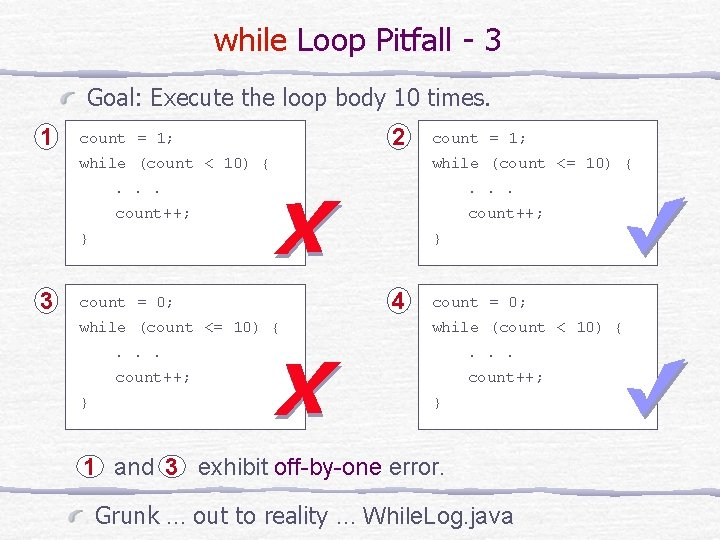while Loop Pitfall - 3 Goal: Execute the loop body 10 times. 1 count = 1; 2 while (count < 10) { while (count <= 10) { . . . count++; } 3 } count = 0; while (count <= 10) { } count = 1; 4 count = 0; while (count < 10) { . . . count++; } 1 and 3 exhibit off-by-one error. Grunk … out to reality … While. Log. java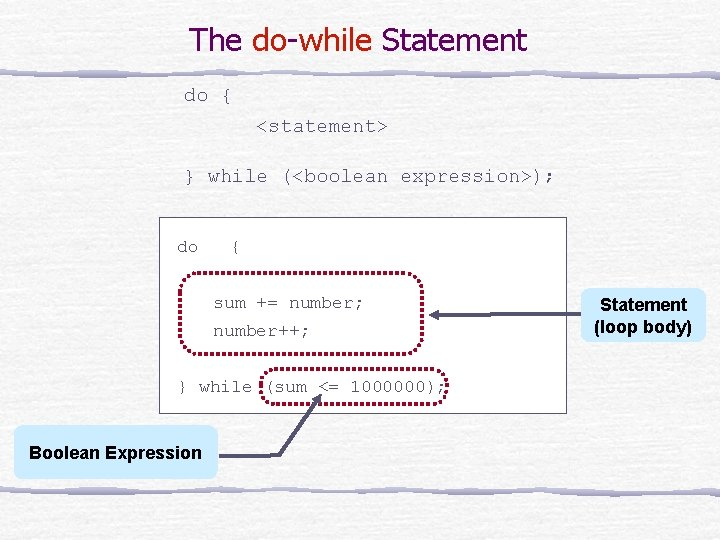The do-while Statement do { <statement> } while (<boolean expression>); do { sum += number; number++; } while (sum <= 1000000); Boolean Expression Statement (loop body)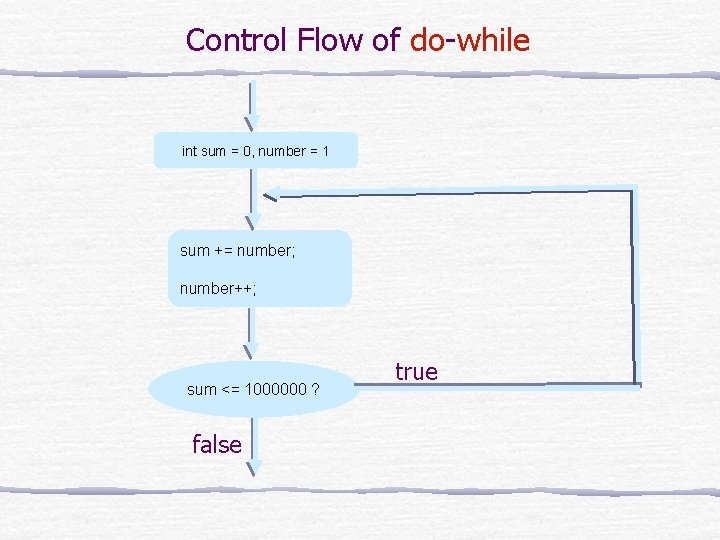Control Flow of do-while int sum = 0, number = 1 sum += number; number++; sum <= 1000000 ? false true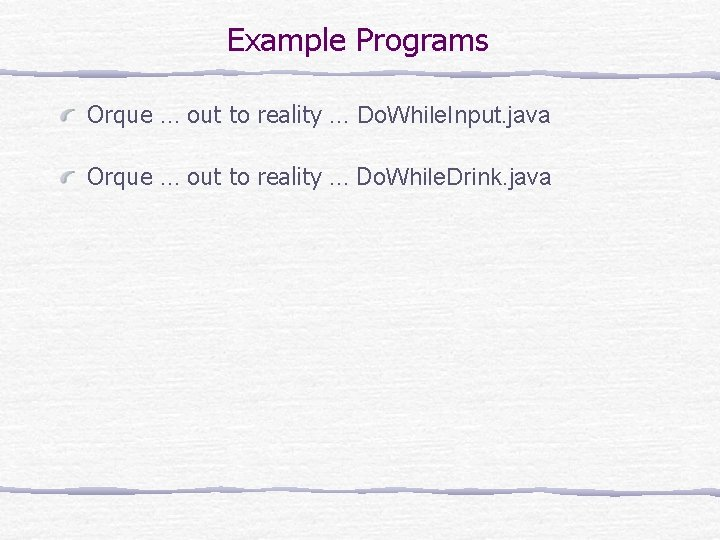Example Programs Orque … out to reality … Do. While. Input. java Orque … out to reality … Do. While. Drink. java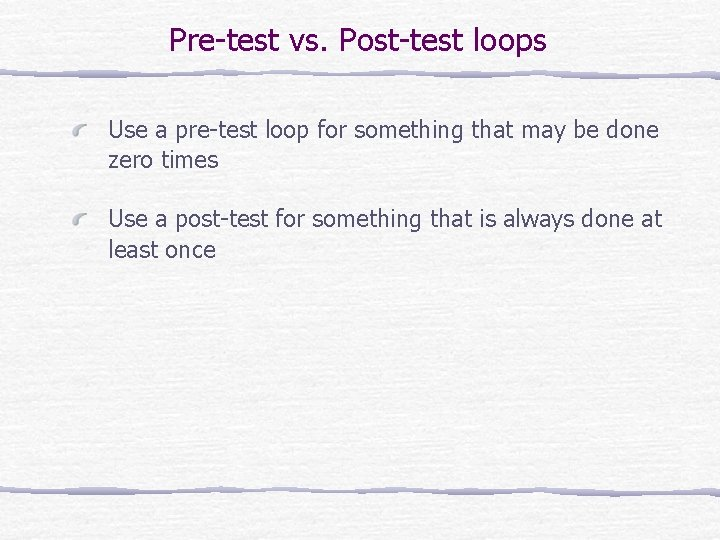Pre-test vs. Post-test loops Use a pre-test loop for something that may be done zero times Use a post-test for something that is always done at least onceThe for Statement for ( <initialization>; <boolean expression>; <update> <statement> int index, sum = 0, number; Boolean Expression Initialization for ( index = 0 ; index < 20 number = keyboard. next. Int(); sum += number; } Update ; index++ ) { Statement (loop body) )Control Flow of for i = 0; false i < 20 ? true number = input. Box. get. Integer( ); sum += number; i ++;Example Programs Poodyplat … out to reality … For. Print. java Poodyplat … out to reality … For. Power. java Poodyplat … out to reality … For. Fibonacci. java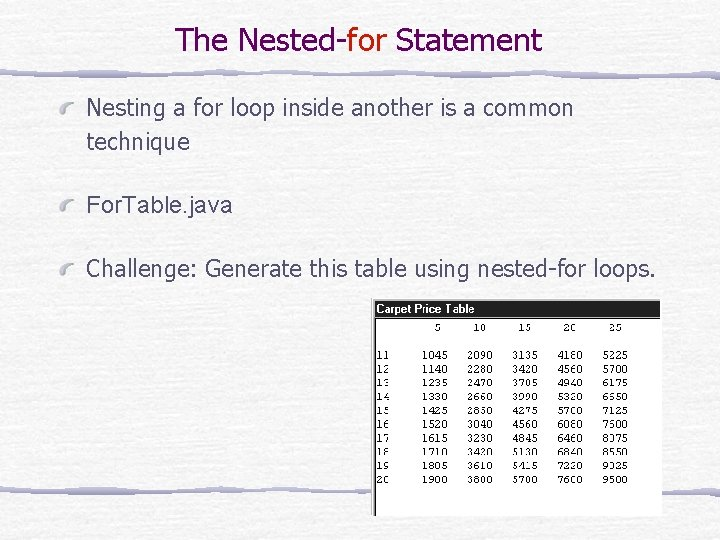The Nested-for Statement Nesting a for loop inside another is a common technique For. Table. java Challenge: Generate this table using nested-for loops.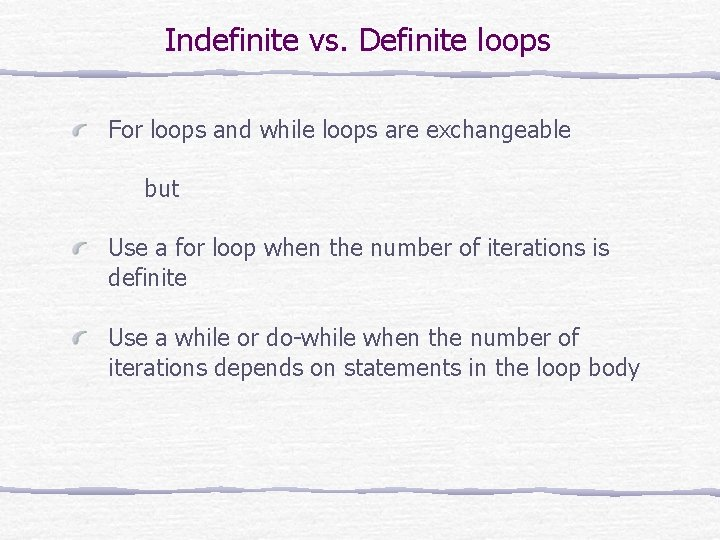Indefinite vs. Definite loops For loops and while loops are exchangeable but Use a for loop when the number of iterations is definite Use a while or do-while when the number of iterations depends on statements in the loop body